The REG Procedure

Polynomial Regression

Consider a response variable Y that can be predicted by a polynomial function of a regressor variable X. You can estimate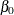, the intercept;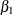, the slope due to X; and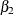, the slope due to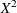, in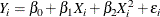for the observations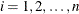.

Consider the following example on population growth trends. The population of the United States from 1790 to 2000 is fit to linear and quadratic functions of time. Note that the quadratic term, YearSq, is created in the DATA step; this is done since polynomial effects such as Year*Year cannot be specified in the MODEL statement in PROC REG. The data are as follows:

data USPopulation;
input Population @@;
retain Year 1780;
Year       = Year+10;
YearSq     = Year*Year;
Population = Population/1000;
datalines;
3929 5308 7239 9638 12866 17069 23191 31443 39818 50155
62947 75994 91972 105710 122775 131669 151325 179323 203211
226542 248710 281422
;

proc reg data=USPopulation plots=ResidualByPredicted;
var YearSq;
model Population=Year / r clm cli;
run;


The DATA option ensures that the procedure uses the intended data set. Any variable that you might add to the model but that is not included in the first MODEL statement must appear in the VAR statement.

The "Analysis of Variance" and "Parameter Estimates" tables are displayed in Figure 97.5.

Figure 97.5: ANOVA Table and Parameter Estimates

The REG Procedure
Model: MODEL1
Dependent Variable: Population

Analysis of Variance
Source DF Sum of
Squares
Mean
Square
F Value Pr > F
Model 1 146869 146869 228.92 <.0001
Error 20 12832 641.58160
Corrected Total 21 159700

 Root MSE R-Square 25.3295 0.9197 94.648 0.9156 26.7617

Parameter Estimates
Variable DF Parameter
Estimate
Standard
Error
t Value Pr > |t|
Intercept 1 -2345.85498 161.39279 -14.54 <.0001
Year 1 1.28786 0.08512 15.13 <.0001

The Model F statistic is significant (F = 228.92, p < 0.0001), indicating that the model accounts for a significant portion of variation in the data. The R-square indicates that the model accounts for 92% of the variation in population growth. The fitted equation for this model is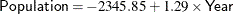In the MODEL statement, three options are specified: R requests a residual analysis to be performed, CLI requests 95% confidence limits for an individual value, and CLM requests these limits for the expected value of the dependent variable. You can request specific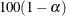% limits with the ALPHA= option in the PROC REG or MODEL statement.

Figure 97.6 shows the "Output Statistics" table. The residual, its standard error, the studentized residual, and Cook’s D are displayed for each observation. The studentized residual is the residual divided by its standard error. Cook’s D is a measure of the change in the predicted values upon deletion of that observation from the data set; hence, it measures the influence of the observation on the estimated regression coefficients. The studentized residuals and Cook’s D statistics are displayed in Figure 97.7.

Studentized residuals follow a beta distribution and can be used to identify outlying or extreme observations:

• Having a few large absolute studentized residuals is no cause for alarm.

• Many observations having absolute studentized residuals greater than two might indicate an inadequate model.

• Absolute studentized residuals greater than three are rarer; they should be regarded as extreme, and the data should be reviewed carefully.

Figure 97.6: Output Statistics

The REG Procedure
Model: MODEL1
Dependent Variable: Population

Output Statistics
Obs Dependent
Variable
Predicted
Value
Std
Error
Mean
Predict
95% CL Mean 95% CL Predict Residual Std Error
Residual
Student
Residual
Cook's D
1 3.93 -40.5778 10.4424 -62.3602 -18.7953 -97.7280 16.5725 44.5068 23.077 1.929 0.381
2 5.31 -27.6991 9.7238 -47.9826 -7.4156 -84.2950 28.8968 33.0071 23.389 1.411 0.172
3 7.24 -14.8205 9.0283 -33.6533 4.0123 -70.9128 41.2719 22.0595 23.666 0.932 0.063
4 9.64 -1.9418 8.3617 -19.3841 15.5004 -57.5827 53.6991 11.5798 23.909 0.484 0.014
5 12.87 10.9368 7.7314 -5.1906 27.0643 -44.3060 66.1797 1.9292 24.121 0.080 0.000
6 17.07 23.8155 7.1470 8.9070 38.7239 -31.0839 78.7148 -6.7465 24.300 -0.278 0.003
7 23.19 36.6941 6.6208 22.8834 50.5048 -17.9174 91.3056 -13.5031 24.449 -0.552 0.011
8 31.44 49.5727 6.1675 36.7075 62.4380 -4.8073 103.9528 -18.1297 24.567 -0.738 0.017
9 39.82 62.4514 5.8044 50.3436 74.5592 8.2455 116.6573 -22.6334 24.655 -0.918 0.023
10 50.16 75.3300 5.5491 63.7547 86.9053 21.2406 129.4195 -25.1750 24.714 -1.019 0.026
11 62.95 88.2087 5.4170 76.9090 99.5084 34.1776 142.2398 -25.2617 24.743 -1.021 0.025
12 75.99 101.0873 5.4170 89.7876 112.3870 47.0562 155.1184 -25.0933 24.743 -1.014 0.025
13 91.97 113.9660 5.5491 102.3907 125.5413 59.8765 168.0554 -21.9940 24.714 -0.890 0.020
14 105.71 126.8446 5.8044 114.7368 138.9524 72.6387 181.0505 -21.1346 24.655 -0.857 0.020
15 122.78 139.7233 6.1675 126.8580 152.5885 85.3432 194.1033 -16.9483 24.567 -0.690 0.015
16 131.67 152.6019 6.6208 138.7912 166.4126 97.9904 207.2134 -20.9329 24.449 -0.856 0.027
17 151.33 165.4805 7.1470 150.5721 180.3890 110.5812 220.3799 -14.1555 24.300 -0.583 0.015
18 179.32 178.3592 7.7314 162.2317 194.4866 123.1163 233.6020 0.9638 24.121 0.040 0.000
19 203.21 191.2378 8.3617 173.7956 208.6801 135.5969 246.8787 11.9732 23.909 0.501 0.015
20 226.54 204.1165 9.0283 185.2837 222.9493 148.0241 260.2088 22.4255 23.666 0.948 0.065
21 248.71 216.9951 9.7238 196.7116 237.2786 160.3992 273.5910 31.7149 23.389 1.356 0.159
22 281.42 229.8738 10.4424 208.0913 251.6562 172.7235 287.0240 51.5482 23.077 2.234 0.511

Figure 97.7: Studentized Residuals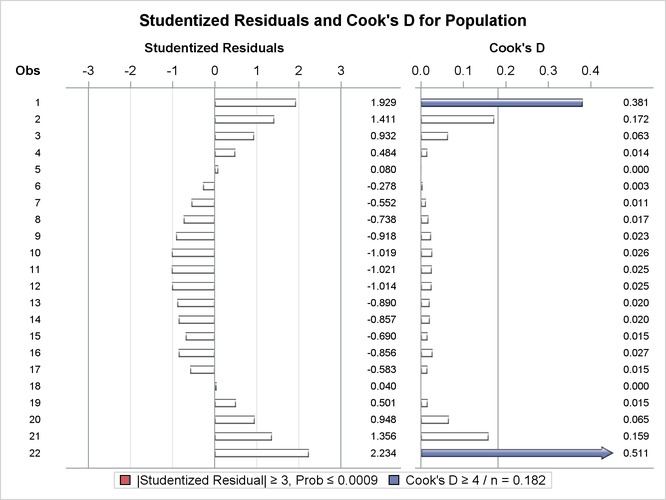Figure 97.8 shows the residual statistics table. A fairly close agreement between the PRESS statistic (see Table 97.7) and the sum of squared residuals indicates that the MSE is a reasonable measure of the predictive accuracy of the fitted model (Neter, Wasserman, and Kutner 1990).

Figure 97.8: Residual Statistics

 Sum of Residuals 0 12832 16662

Graphical representations are very helpful in interpreting the information in the "Output Statistics" table. When ODS Graphics is enabled, the REG procedure produces a default set of diagnostic plots that are appropriate for the requested analysis.

Figure 97.9 displays a panel of diagnostics plots. These diagnostics indicate an inadequate model:

• The plots of residual and studentized residual versus predicted value show a clear quadratic pattern.

• The plot of studentized residual versus leverage seems to indicate that there are two outlying data points. However, the plot of Cook’s D distance versus observation number reveals that these two points are just the data points for the endpoint years 1790 and 2000. These points show up as apparent outliers because the departure of the linear model from the underlying quadratic behavior in the data shows up most strongly at these endpoints.

• The normal quantile plot of the residuals and the residual histogram are not consistent with the assumption of Gaussian errors. This occurs as the residuals themselves still contain the quadratic behavior that is not captured by the linear model.

• The plot of the dependent variable versus the predicted value exhibits a quadratic form around the 45-degree line that represents a perfect fit.

• The "Residual-Fit" (or RF) plot consisting of side-by-side quantile plots of the centered fit and the residuals shows that the spread in the residuals is no greater than the spread in the centered fit. For inappropriate models, the spread of the residuals in such a plot is often greater than the spread of the centered fit. In this case, the RF plot shows that the linear model does indeed capture the increasing trend in the data, and hence accounts for much of the variation in the response.

Figure 97.9: Diagnostics Panel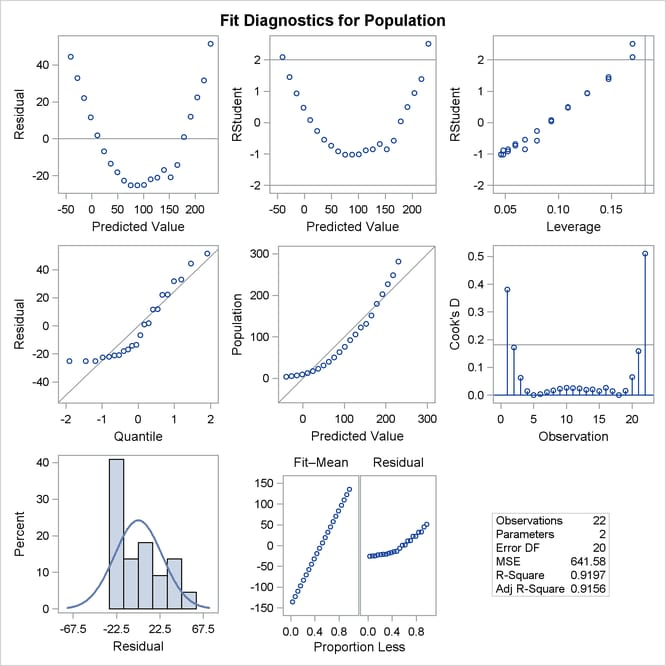Figure 97.10 shows a plot of residuals versus Year. Again you can see the quadratic pattern that strongly indicates that a quadratic term should be added to the model.

Figure 97.10: Residual Plot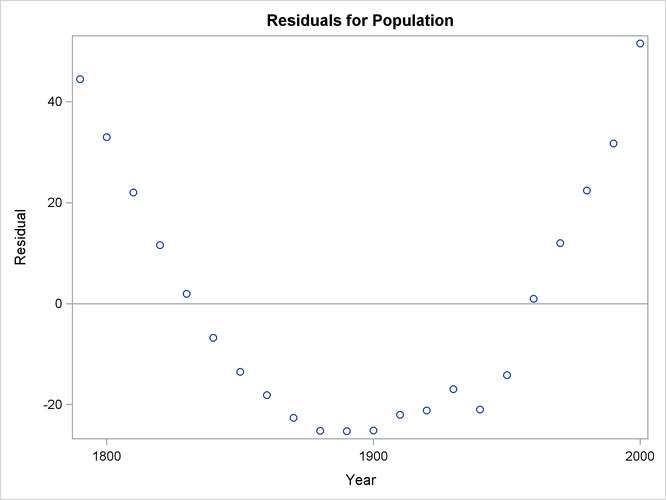Figure 97.11 shows the FitPlot consisting of a scatter plot of the data overlaid with the regression line, and 95% confidence and prediction limits. Note that this plot also indicates that the model fails to capture the quadratic nature of the data. This plot is produced for models containing a single regressor. You can use the ALPHA= option in the model statement to change the significance level of the confidence band and prediction limits.

Figure 97.11: Fit Plot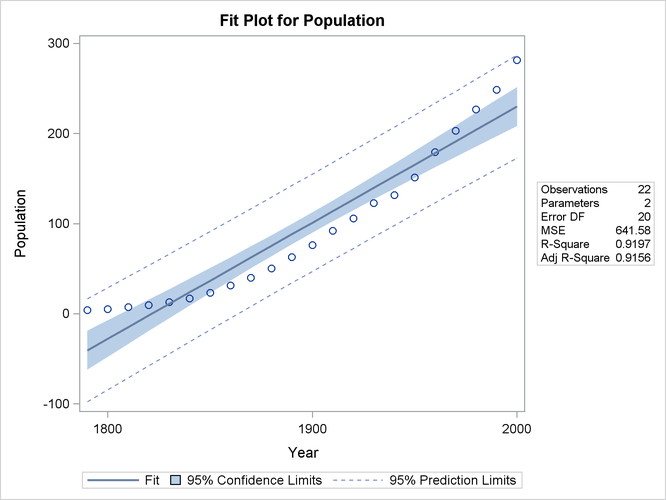These default plots provide strong evidence that the Yearsq needs to be added to the model. You can use the interactive feature of PROC REG to do this by specifying the following statements:

   add YearSq;
print;
run;


The ADD statement requests that YearSq be added to the model, and the PRINT command causes the model to be refit and displays the ANOVA and parameter estimates for the new model. The print statement also produces updated ODS graphical displays.

Figure 97.12 displays the ANOVA table and parameter estimates for the new model.

Figure 97.12: ANOVA Table and Parameter Estimates

Analysis of Variance
Source DF Sum of
Squares
Mean
Square
F Value Pr > F
Model 2 159529 79765 8864.19 <.0001
Error 19 170.97193 8.99852
Corrected Total 21 159700

 Root MSE R-Square 2.99975 0.9989 94.648 0.9988 3.16938

Parameter Estimates
Variable DF Parameter
Estimate
Standard
Error
t Value Pr > |t|
Intercept 1 21631 639.50181 33.82 <.0001
Year 1 -24.04581 0.67547 -35.60 <.0001
YearSq 1 0.00668 0.00017820 37.51 <.0001

The overall F statistic is still significant (F = 8864.19, p < 0.0001). The R-square has increased from 0.9197 to 0.9989, indicating that the model now accounts for 99.9% of the variation in Population. All effects are significant with p < 0.0001 for each effect in the model.

The fitted equation is now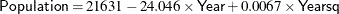Figure 97.13 show the panel of diagnostics for this quadratic polynomial model. These diagnostics indicate that this model is considerably more successful than the corresponding linear model:

• The plots of residuals and studentized residuals versus predicted values exhibit no obvious patterns.

• The points on the plot of the dependent variable versus the predicted values lie along a 45-degree line, indicating that the model successfully predicts the behavior of the dependent variable.

• The plot of studentized residual versus leverage shows that the years 1790 and 2000 are leverage points with 2000 showing up as an outlier. This is confirmed by the plot of Cook’s D distance versus observation number. This suggests that while the quadratic model fits the current data well, the model might not be quite so successful over a wider range of data. You might want to investigate whether the population trend over the last couple of decades is growing slightly faster than quadratically.

Figure 97.13: Diagnostics Panel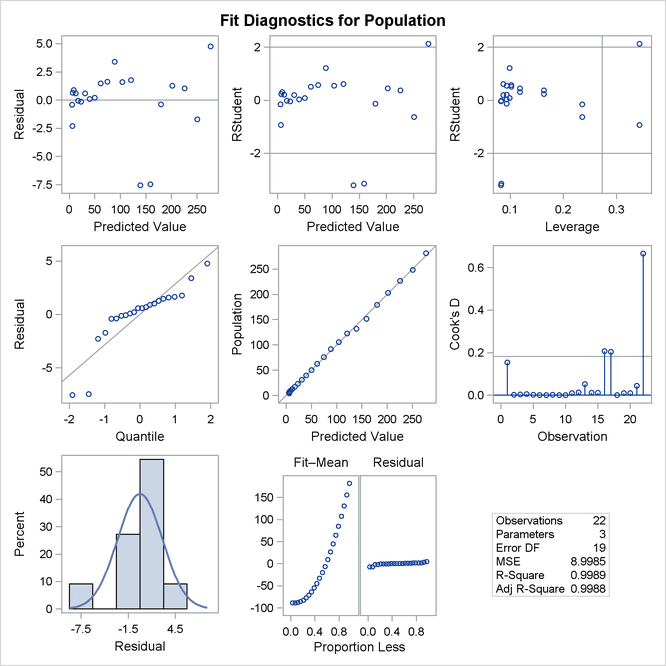When a model contains more than one regressor, PROC REG does not produce a fit plot. However, when all the regressors in the model are functions of a single variable, it is appropriate to plot predictions and residuals as a function of that variable. You request such plots by using the PLOTS=PREDICTIONS option in the PROC REG statement, as the following code illustrates:


proc reg data=USPopulation plots=predictions(X=Year);
model Population=Year Yearsq;
quit;

ods graphics off;


Figure 97.14 shows the data, predictions, and residuals by Year. These plots confirm that the quadratic polynomial model successfully model the growth in U.S. population between the years 1780 and 2000.

Figure 97.14: Predictions and Residuals by Year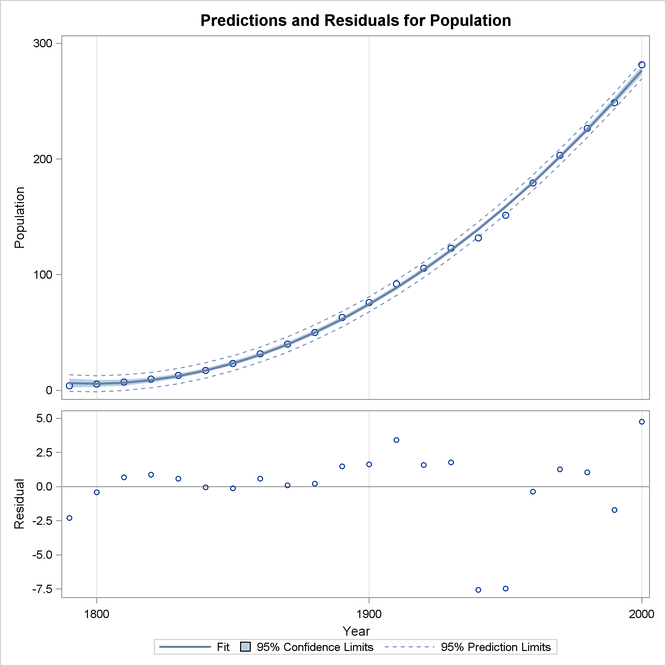To complete an analysis of these data, you might want to examine influence statistics and, since the data are essentially time series data, examine the Durbin-Watson statistic.